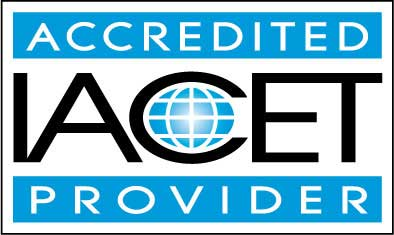# Course Syllabus## Course Description

An understanding of algebra is a foundational skill to virtually all topics in higher-level mathematics, and it is useful in science, statistics, accounting, and numerous other professional and academic areas. This course builds a bridge between simple whole-number arithmetic operations, such as addition, subtraction, multiplication, and division, and algebra.

This course begins by reviewing the basic arithmetic operations and their mutual relationships, and it then goes on to develop an understanding of fractions and how to perform operations on them. Exponents and their properties are then derived, and radicals (and other roots) are presented.

This course shows how exponents and roots are related, as well as how these are related to multiplication and division. Throughout this first portion of the course, the use of symbols in place of numbers is introduced and developed, leading to an investigation into variables and algebraic relations in the latter portion of the course.

This course also describes how to graph algebraic relations and how to solve simple linear equations. Finally, the course applies these various concepts to geometry by considering some of the characteristics of triangles, rectangles, and circles, and it also applies them to basic probability and statistics.

Thus, this course not only provides a fundamental set of algebra skills, but it demonstrates how these skills can be used to study various problems that have real-world applications.
• Completely Online
• Self-Paced
• 6 Months to Complete
• Start Anytime
• PC & Mac Compatible
• Android & iOS Friendly
• Accredited CEUs## Learning Outcomes

By successfully completing this course, students will be able to:
• Describe basic operations or numbers and signs.
• Solve simple to complex fraction problems.
• Solve problems involving decimals, percents, and ratios.
• Solve problems involving exponents.
• Summarize variables and algebraic relations.
• Graph algebraic relations.
• Solve linear equations.
• Solve problems involving basic geometry, rectangles and triangles.
• Solve probability and statistics problems.
• Demonstrate mastery of lesson content at levels of 70% or higher.

## Assessment Guide

Assessment Points
Introduction Assignment 1 points
Lesson 1 - Review of Basic Operations 10 points
Lesson 1 Exercises 7 points
Lesson 2 – Fractions I 10 points
Lesson 2 Exercises 9 points
Lesson 3 – Fractions II 10 points
Lesson 3 Exercises 8 points
Lesson 4 – Decimals, Percents, and Ratios 10 points
Lesson 4 Exercises 10 points
Lesson 5 – Exponents I 10 points
Lesson 5 Exercises 8 points
Lesson 6 – Exponents II 10 points
Lesson 6 Exercises 9 points
Lesson 7 – Radicals I 10 points
Lesson 7 Exercises 8 points
Lesson 8 – Radicals II 10 points
Lesson 8 Exercises 6 points
Lesson 9 Variables and Algebraic Relations 10 points
Lesson 9 Exercises 4 points
Lesson 10 – Graphing Algebraic Relations 10 points
Lesson 11 Solving Linear Equations 10 points
Lesson 11 Exercises 4 points
Lesson 12 – Geometry I: Rectangles and Triangles 10 points
Lesson 12 Exercises 3 points
Lesson 13 – Geometry II 10 points
Lesson 13 Exercises 4 points
Lesson 14 Introduction to Probability 10 points
Lesson 14 Exercises 5 points
Lesson 15 Introduction to Statistics 9 points
The Final Exam 220 points
Lesson 15 Exercises 6 points